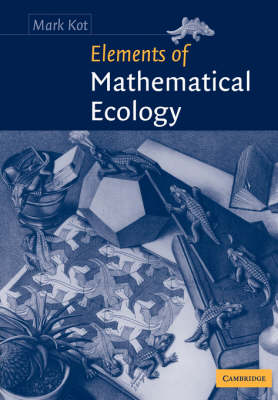•Elements of Mathematical Ecology (Paperback)

(author)
£73.99
Paperback 464 Pages / Published: 19/07/2001
• We can order this from the publisher

Usually dispatched within 10 working days

Elements of Mathematical Ecology provides an introduction to classical and modern mathematical models, methods, and issues in population ecology. The first part of the book is devoted to simple, unstructured population models that ignore much of the variability found in natural populations for the sake of tractability. Topics covered include density dependence, bifurcations, demographic stochasticity, time delays, population interactions (predation, competition, and mutualism), and the application of optimal control theory to the management of renewable resources. The second part of this book is devoted to structured population models, covering spatially-structured population models (with a focus on reaction-diffusion models), age-structured models, and two-sex models. Suitable for upper level students and beginning researchers in ecology, mathematical biology and applied mathematics, the volume includes numerous clear line diagrams that clarify the mathematics, relevant problems thoughout the text that aid understanding, and supplementary mathematical and historical material that enrich the main text.

Publisher: Cambridge University Press
ISBN: 9780521001502
Number of pages: 464
Weight: 730 g
Dimensions: 244 x 170 x 24 mm

MEDIA REVIEWS
'Kot's current compilation will prove extremely useful ...'. Acta Biotheoretica
'Kot's book fills the need for a rigorous, graduate-level textbook in mathematical population ecology, and does it very well'. Quarterly Review of Biology
'This is a further important book in the field of the theoretical ecology ... the volume will prove invaluable to all students of mathematical biology and theoretical ecology.' Folia Geobotanica
'... suitable for a year's course of study for upper level students and beginning researchers in ecology, mathematical biology and applied mathematics. ... an excellent introduction to mathematical ecology for the reader with an appropriate mathematical background (calculus, differential equations and probability theory).' Zentralblatt MATH